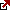# What is Equivalent Annual Annuity?

What is an Equivalent Annual Annuity?

The equivalent annual annuity or EAA represents the capital budgeting method that calculates the constant annual cash flow generated by a project over its lifespan. In simple words,  EAA shows the net present value of an investment as a series of equal cash flows produced by a project over its lifetime. This method evaluating projects with different life durations.

What equivalent annual annuity means? Equivalent Annual Annuity or EAA means in practice a method evaluating projects with different life durations. Specific traditional metrics can also determine financial projects’ overall viability to assess the projects’ profitability like IRR, Payback period or NPV, etc. However, finding out the financial efficiency of projects equivalent to the annual annuity is the right metric.

How to calculate equivalent annual annuity?

To explain the present net value of a sequence of investments for a certain period, equal cash formula flow is used in capital budgeting to calculate EAA. The formula is

C = (r x NPV) / (1 – (1 + r)^-n )

Where:

R is the discount rate of the project.

NPV is the Net present value of the cash flows of the project

n is the life of the project in years

The current worth of an investment with irregular flows of cash is used as NPV in the formula. The investment period or n’ is not considered while using NPV to compare two investments in different projects. The NPV of the financing for 15 years will be higher than the investment for a period of 4 years. An equivalent annual annuity formula can help restore the investment period by showing its NPV as a sequence of cash flow.

How to calculate equivalent annual annuity in Excel?

The usefulness of the formula of EAA

What does the equivalent annual annuity tell us?
The formula of the EAA can be useful to compare two projects with the same NPV but with a different life; the term says 15 years and 4 years. The project with 4 years life term will show a higher flow of cash in the formula of EAA as the return will be received sooner by it than the project with 15 years life term. This formula should be applied while distinguishing two real-life investments, as it may not be possible otherwise.

The formula of EAA can also be useful while reinvesting in a project. While using this formula to evaluate the possibilities of reinvestment in a project with a short life term, the investment income will not be considered. The reinvestment with similar earnings will not be regarded as the present investment while using this formula for comparing the EAA of two projects based on their life term.

Derivation of the formula of the Equivalent Annual Annuity

The formula of the EAA has been derived from the equation of the annuity payment for a given value. The relevance of using the method of EAA is based on the replacement of present value with NPV or net present worth.

Example for using the formula of Equivalent Annual Annuity

Suppose there are two machines – machine A, a machine to mix the dough, and machine B, an icing machine. Let the life span of machine A is 6 years, and that of machine B is 4 years. Now the owner of a doughnut shop wants to buy one of these machines. So he will compare their equivalent annual annuity by using NPV and the life span of each machine. Suppose the NPV of machine A is \$4 million and Machine B is \$3 million, and the cost of capital of doughnut shop is 10%. The EAA of each device will be calculated as under

Based on the EAA of both the machines, the doughnut shop should invest in machine B due to its higher EAA.

Latest posts by Fxigor (see all)

Fxigor

Igor has been a trader since 2007. Currently, Igor works for several prop trading companies. He is an expert in financial niche, long-term trading, and weekly technical levels. The primary field of Igor's research is the application of machine learning in algorithmic trading. Education: Computer Engineering and Ph.D. in machine learning. Igor regularly publishes trading-related videos on the Fxigor Youtube channel. To contact Igor write on: igor@forex.in.rs

Trade gold and silver. Visit the broker's page and start trading high liquidity spot metals - the most traded instruments in the world.Diversify your savings with a gold IRA.

Gold & silver portfolio-building assistance from product professionals.

If you want to trade stocks try: